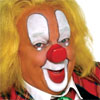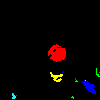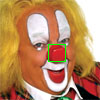# Pure Ruby colored blob detection

2011-04-11

Over the last few weeks, I’ve become increasingly interested in computer vision. After finally getting OpenCV running, playing around with a little face detection and searching for solutions to weird problems that occurred, I decided to dive a bit deeper into computer vision to figure out how it actually works.I started out with blob detection, which simply means detecting, locating and measuring an object in an image. A really simple way of doing something like that (without having to worry about object textures and creepy stuff like that), is to use color to identify blobs. In this article, we’re going to build a clown’s nose detector by finding the biggest red blob in an image. We won’t use any computer vision libraries, the only thing we’ll need is ChunkyPNG by @wvanbergen (but you can use any library that allows you to loop over a image’s pixels).Here’s the image we’ll be working with. If you’re interested, it’s Bassie from the dutch circus duo Bassie & Adriaan. The important thing about this image is that the nose is the biggest red object, since we won’t really be recognizing things as clowns’ noses, we’re just detecting red objects.

### Binary images

First, we load in the image and simplify it to make it easier to work with. Since we’re only interested in the red pixels, we can turn the original image into a binary one. We go over each of the original image’s pixels and figure out it’s redness. If the pixel is red enough (we’ll use a threshold of 100/255), we give it a value of -1, meaning the pixel is interesting but we still have to process it. We don’t need to do anything with the other pixels, so we’ll give those a value of 0, these are the background pixels.

``````require 'chunky_png'

image = ChunkyPNG::Image.from_file('bassie.png')
working_image = image.dup

working_image.pixels.map! do |pixel|
redness = ChunkyPNG::Color.r(pixel) - (ChunkyPNG::Color.g(pixel) + ChunkyPNG::Color.b(pixel))
redness > 100 ? -1 : 0
end
``````

Here’s the working image with the blobs in white and the background pixels in black:Now we have an image we can work with, let’s divide the separate blobs so we can measure them individually to figure out which one the nose is. To do this, we’ll loop over every pixel in the image and see if it has a -1 value because it still needs to be processed. If it does, we’ll give it a label and add it to an `areas` array.

``````areas, label = {}, 0

working_image.height.times do |y|
working_image.row(y).each_with_index do |pixel, x|
(areas[label += 1] ||= []) << [x,y] if pixel == -1
end
end
``````

As you probably figured out already, we’re assigning each pixel with a -1 value a separate label right now. This means pixels in the same blob get different labels. We don’t want that, since we want to label groups of pixels that belong to the same blob. To do that, we need to check the pixel’s neighbors too.

### Pixel neighborhoods

To be able to find connected pixels, we need to know each pixel’s neighborhood. For this example we’ll use a 4-neighborhood, which means we use the pixels right above (north), to the right (east), right below (south) and to the left (west) of the current one. The 8-neighborhood also uses the northeast, southeast, southwest and northwest pixels, but we won’t really need that for something simple like this.

We need to keep in mind that some pixels don’t have four neighbors, like the one in the top left, which doesn’t have any north or west neighbors because they would fall out of the image. Luckily, ChunkyPNG has some nice methods to help us figure out if pixels actually exist. We’ll implement a `neighbors` method directly into `ChunkyPNG::Image`:

``````  class ChunkyPNG::Image
def neighbors(x,y)
[[x, y-1], [x+1, y], [x, y+1], [x-1, y]].select do |xy|
include_xy?(*xy)
end
end
end
``````

Using our new neighborhood method, we can improve the blob separator we wrote earlier. We implement a method named `label_recursively`, which assigns a label to the current pixel, adds the current pixel to the `areas` array, checks its neighbors and calls itself (without changing the label) on the neighbors if their values are -1 too. When none of the neighbors’ values are -1, the loop will stop until the main loop finds another -1-pixel. If that happens, the label gets increased by 1 and the `label_recursively` method is called again:

``````def label_recursively(image, areas, label, x, y)
image[x,y] = label
(areas[label] ||= []) << [x,y]

image.neighbors(x,y).each do |xy|
if image[*xy] == -1
areas[label] << xy
label_recursively(image, areas, label, *xy)
end
end
end

areas, label = {}, 0

working_image.height.times do |y|
working_image.row(y).each_with_index do |pixel, x|
label_recursively(working_image, areas, label += 1, x, y) if pixel == -1
end
end
``````

The `areas` array holds the blobs as labeled groups of pixels now. If we would color the detected blobs, it would look somewhat like this:As you can see, there were some detected areas that overlap and should have been counted as one. This shouldn’t be a problem for this example, but using an 8-neighborhood can solve some of these issues and make the result more precise, if you end up needing it.

All we have to do to detect the nose is find out which area is the biggest (which one has the most pixels). Since we stored the areas in the `areas` array, this should be straightforward:

``````area = areas.values.max { |result, area| result.length <=> area.length }
``````

Now, `area` holds an array of the pixels in the blob we’re interested in. Since we want to put a bounding box around the blob we found, we get the highest and lowest x and y values, create a rectangle on the image using ChunkyPNG’s drawing tools and save the image:

``````x, y = area.map{ |xy| xy }, area.map{ |xy| xy }

image.rect(x.min, y.min, x.max, y.max, ChunkyPNG::Color.rgb(0,255,0))
image.save('bassie_detected.png')
``````

And there you go! We successfully detected, located and measured the biggest red blob in the image:Of course, this method isn’t fool proof, but it’s a really simple first step into computer vision. As always, if you used this idea to build something yourself, know of a way to improve the code or have some questions or tips, be sure to let me know. You can find the complete source in this Gist. Have fun!